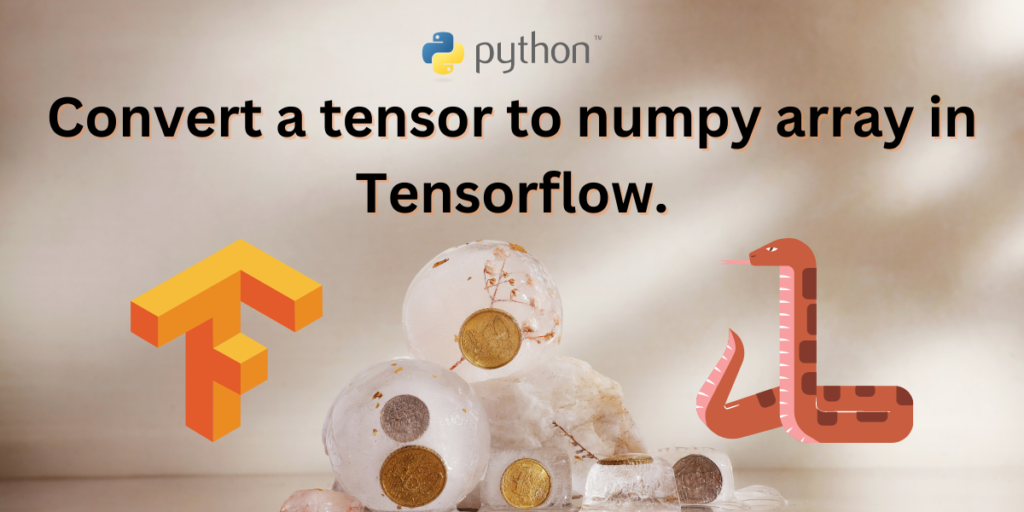# Convert A Tensor To Numpy Array In Tensorflow-ImplementationTensors in python are multi dimensional arrays similar to numpy arrays. They are used as Data containers or data storage units in python. Tensors have a uniform data type.

Tensors are also immutable in nature, that is, once the values are assigned they cannot be re-done. In this aspect, tensors are similar to python strings and tuples which are also immutable in nature.

A generalized vector or matrix, tensors are extensively used in deep learning.

## Applications of Tensors in Deep Learning and Data Science

Tensors are used for a variety of reasons. Some of them are:

• Tensors are extensively used to store matrices and vectors in deep learning algorithms.
• They are also used in linear algebraic methods and complex scientific calculations.
• Arithmetic operations can be performed on tensors easily.
• Just like matrix operations, such as ,matrix addition, subtraction, multiplication and division can also be performed using tensors.
• They are used for various mapping purposes such as image mapping and data structures and videos and photos.

## What is TensorFlow?

Tensorflow is a open source library provided by google for machine learning purposes. It is used to load and manipulate data. It is also used for training artificial intelligence and deep learning models.

Tensorflow is a platform that is also used in developing and training neural networks.

## Comparing Tensors in TensorFlow and NumPy Arrays

The main difference between numpy arrays and tensors in tensorflow, is that tensors are faster than numpy array when processing speeds are compared. Tensors are supported by accelerated GPU which is not supported by NumPy.

Tensorflow is an open source API that can perform gradient computations unlike numpy arrays. Numpy is the official scientific computational library supported by python.

There is syntactical difference between numpy functions and tensorflow functions. The numpy function call are written as INPUT.reshape(3,3) whereas in tensorflow the same thing is written as tf.reshape(INPUT,(3,3)).

## Installing Required Libraries and Ensuring Compatibility

Before we began using the above mentioned APIs and libraries, we need to make sure that they are on our computers, all installed and updated. Run the following code to make sure you have all the basic requirements met:

```pip install numpy
pip install tensorflow
```

Note: Make sure you have the latest version of pip, python and tensorflow in your system as it is easier to implement the conversion of tensors to numpy arrays in the updated version. The updated version by default has eager execution enabled, which will carry out the evaluation of operations immediately without any trouble. This was not the case in version=1.0 of tensorflow, which is why the method in the given sections will only work on updated versions of tensorflow.

It is recommended to use the latest version to avoid unwanted errors and problems.

Related: Check if Python Package is installed.

## Code Implementation for Converting Tensors to NumPy Arrays in TensorFlow

To convert a tensor to a NumPy array in TensorFlow, first import the TensorFlow library. Create a tensor using ‘tf.constant’. To convert the tensor into a NumPy array, use the ‘numpy()’ method by calling ‘tensor.numpy()’. This will return a NumPy array with the same values and shape as the original tensor. Let’s look at how we can convert a tensor into a numpy array in tensorflow easily.

```#importing required modules
import tensorflow as tf
#creating a tensor in the variable called val
val = tf.constant([[12, 22], [32, 42],[52,62],[72,82],[92,102]])
#dispplaying the tensor with its details
print("The tensor is= ",val)
#transforming the tensor and displaying the result
print("The transformed tensor into a numpy array is= ", val.numpy())
```

The output would be:

```The tensor is=  tf.Tensor(
[[ 12  22]
[ 32  42]
[ 52  62]
[ 72  82]
[ 92 102]], shape=(5, 2), dtype=int32)
The transformed tensor into a numpy array is=  [[ 12  22]
[ 32  42]
[ 52  62]
[ 72  82]
[ 92 102]]
```

Suggested : Introduction to Python Modules.

## Converting Tensors to NumPy Arrays in TensorFlow 1.0

Using older version of tensorflow can result in attribute errors hence it is not advisable to use older versions. Use the previous method (preferably). If for some reason, you have to inevitably use this version(that is version=1.0) we have provided a possible work around the errors below:

```#importing tensorflow version 1.0
import tensorflow.compat.v1 as tf
tf.compat.v1.disable_v2_behavior()
#creating a tensor
tensor_org = tf.constant([[13,23,33], [43,53,63]])
#displaying original type of tensor
print(type(tensor_org))
#converting the tensor into numpy array and displaying the datatype after conversion.
tensor_newarray = tensor_org.eval(session=tf.Session())
print(type(tensor_newarray))
```

The output I got was:

```WARNING:tensorflow:From /usr/local/lib/python3.9/dist-packages/tensorflow/python/compat/v2_compat.py:107: disable_resource_variables (from tensorflow.python.ops.variable_scope) is deprecated and will be removed in a future version.
Instructions for updating:
non-resource variables are not supported in the long term
<class 'tensorflow.python.framework.ops.Tensor'>
<class 'numpy.ndarray'>
```